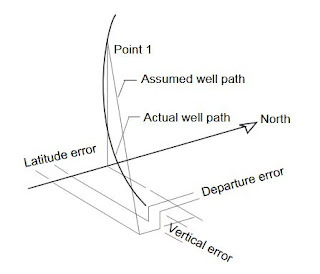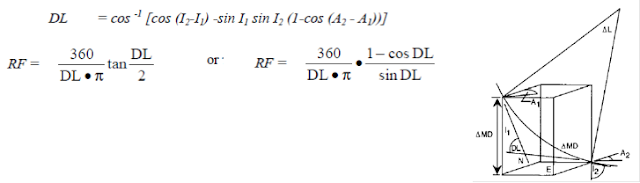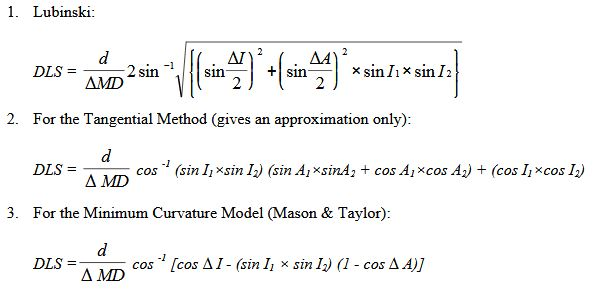# DIRECTIONAL DRILLING SURVEY CALCULATION METHODS

## Directional Drilling Survey Calculations Methods

### Introduction To Directional Survey Calculations Methods

First these article is to understand how the directional survey calculations goes. Now a days you will find a lot of soft-wares for the directional survey calculations. In the same time I have found a good directional survey calculations excel sheet which will help you in your calculations.
Regardless of which conventional directional survey method is used (single-shot, multishot, steering tool, surface readout gyro, MWD), the following three pieces of information are known at the end of a successful directional survey:
• Survey Measured Depth
• Borehole Inclination
• Borehole Azimuth (corrected to relevant North).
In order to be sure the latest bottom-hole position, it is necessary to perform the directional survey calculation which includes the three inputs listed above. Only then can the latest bottom-hole coordinates be plotted on the directional well plot (TVD vs Vertical Section on the vertical plot, N/S vs E/W rectangular coordinates on horizontal plot). Projections to the target, etc., can then be done (See below Figure).

A number of directional survey calculation methods have been used in directional drilling. Of these,only four have had widespread use:
• Tangential calculation method.
• Average Angle calculation method.
• Radius of Curvature calculation method.
• Minimum Curvature calculation method.
The Tangential Calculation Method is the oldest, least sophisticated and most inaccurate directional survey calculation method.This method should never be used.

Average Angle and Radius of Curvature survey calculations methods are in common field use. Average Angle method (in particular) lends itself easily to a hand-held calculator. Radius of Curvature method is more widely used. However, official directional survey reports should not use either if the above methods except when demanded by the customer.

Minimum Curvature survey calculation method should be used for all office calculations and official survey reports. Where possible, it should also be the field calculation method chosen. The directional driller is advised to have at the well-site a hand-held calculator which is programmed for both Radius of Curvature and Minimum Curvature methods of directional survey calculation.

### Purpose Of Directional Survey

• Orient deflection tools for navigating well paths
• Determine the exact bottom hole location to monitor reservoir performance
• Monitor the actual well path to ensure the target will be reached
• Fulfill requirements of regulatory agencies, such as the Minerals Management Service (MMS) in the U.S
• Calculate the TVD of the various formations to allow geological mapping
• Ensure that the well does not intersect nearby wells
• Evaluate the Dog Leg Severity (DLS), which is the total angular inclination and azimuth in the wellbore, calculated over a standard length (100 ft or 30 m)

Definitions"O" is the Reference Point for the Well.
"S" is the Surface Location Reference Point.
"B" is a Survey Point.
"a" is the Azimuth in degrees of the Vertical Section plane. It is measured in a Horizontal Plane from the North Direction (geographic), beginning at 0° and continuing through 360° (clockwise from North axis).
"TVD" is the projection of SB (Measured Depth, MD, along the well path) onto the vertical axis "z". The distance is SB3.
"HD" is the Horizontal Displacement, measured in the Horizontal plane passing through the Survey Point. The distance is BB3 (between Survey point end “z” axis).
"VS" is the Vertical Section; it is the length of the projection of the Horizontal Displacement (HD) onto the Vertical Section plane defined by its azimuth. The distance is B3B2.
“A Vertical Section Plane” is defined by its Azimuth and the U.S. origin point. Usually, the Vertical Section passing through the center of the Target is used for plotting the well profile.

### Directional Drilling Survey Calculations methods

As mentioned above that no one now do these calculations by hand. but to be able to do the directional survey calculations you will have to understand the methods. Also below after the explanation of these methods, you will find the directional survey calculations excel sheet to be able to download.
These methods have been used in directional drilling. Of these, only four have had widespread use:

####Tangential Survey Calculations Method

This method uses the inclination and hole direction at the lower end of the course length to calculate a straight line representing the wellbore that passes through the lower end of the course length. Because the wellbore is assumed to be a straight line throughout the course length, it is the most inaccurate of the methods discussed and should be abandoned completely.

∆North = ∆ MD sin I2 cos A2
∆East = ∆ MD sin I2 sin A2
∆TVD = ∆ MD cos I2
∆ Displacement = ∆ MD sin I2

#### Balanced tangential Calculations Method

Modifying the tangential directional survey calculation method by taking the direction of the top station for the first half of the course length, then that of the lower station for the second half can substantially reduce the errors in that method. This modification is known as the balanced-tangential method.

This method is very simple to program on hand-held calculators and in spreadsheets and gives accuracy comparable to the minimum-curvature method.
∆North =1/2 x ∆MD (sin I1 cos A1 + sin I2 cos A2)
∆East = 1/2 x ∆MD (sin I1 sin A1 + sin I2 sin A2)
∆TVD = 1/2 x ∆MD (cos I1 + cos I2)
∆ Displacement = 1/2 x ∆MD (sin I1 + sin I2)

#### Average angle Calculation MethodThe directional survey calculation method uses the average of the inclination and hole-direction angles measured at the upper and lower ends of the course length. The average of the two sets of angles is assumed to be the inclination and the direction for the course length. The well path is then calculated with simple trigonometric functions.

∆North = ∆ MD sin A cos B
∆East = ∆ MD sin A sin B
∆TVD = ∆ MD cos A
∆ Displacement (CD) = ∆ MD sin A
∆ Vertical Section = CD x cos ( B - target direction)
Where:
A = (I1+I2)/2
B = (A1+A2)/2

#### Radius of Curvature survey Calculation Method. “is more widely used.”

With the inclination and hole direction measured at the upper and lower ends of the course length, this directional survey calculation method generates a circular arc when viewed in both the vertical and horizontal planes. Curvature radius is one of the most accurate methods available.Minimum Curvature.” should be used for all office calculations and official directional survey” reports

Like the curvature-radius method, this method, the most accurate of all listed, uses the inclination and hole direction measured at the upper and lower ends of the course length to generate a smooth arc representing the well path.

The difference between the curvature-radius and minimum-curvature directional survey calculations methods is that curvature radius uses the inclination change for the course length to calculate displacement in the horizontal plane (the true vertical depth [TVD] is unaffected), whereas the minimum-curvature method uses the Dogleg Severity (DLS) to calculate displacements in both planes.

Minimum curvature is considered to be the most accurate method, but it does not lend itself easily to normal, hand-calculation procedures.#### Mercury Survey Calculation Method

So called because it was used at Mercury, Nevada at the U. S. nuclear test site. This directional survey calculation method combines the tangential and balanced tangential calculation methods, and takes into account the length of the survey tool (STL).It treats the portion of the course over the length of the survey tool as a straight line (i.e.tangential) and the rest of the course in a balanced tangential manner.

∆TVD = (∆MD-STL) /2 × (cos I1+cos I2)+STL × cos I2
∆North = (∆MD-STL) /2 × (sin I1+cos A1+sin I2 cos A2)+ STL × sin I2 cos A2
∆East = (∆MD-STL) /2 × (sin I1+sin A1+sin I2 sin A2)+ STL × sin I2 sin A2

Comparison Between Diffeernt Directional Survey Calculations Methods

The directional survey results are compared against those from the minimum-curvature method, as shown in Below Table. Large errors are seen in the tangential method for only approximately 1,900 ft of deviation. This demonstrates that the tangential method is inaccurate and should be abandoned completely. The balanced-tangential and average-angle methods are more practical for field calculations and should be used when sophisticated computational equipment or expertise may not be available. These should be noted as “Field Results Only.”

### Dogleg severity

Dogleg Severity is a measure of the amount of change of inclination and/or direction of a bore hole. It is usually expressed in degrees per 100 feet or degrees per 10 or 30 metres of course length.

Several formulae are available to compute the total effects when there is a change in both inclination and direction between survey points. In the following formulae:

d = DLS
Interval I1 = Inclination at Survey point 1
A1 =Azimuth at Survey point 1
I2 =Inclination at Survey point 2
A2 =Azimuth at Survey point 2

The following formula is commonly used in estimating fatigue and strength criteria for tubular goods. It makes no assumptions about the well path, and is therefore independent of survey calculation methods.